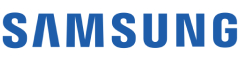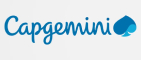New update is available. Click here to update.

# Majority Element lll

Medium0/80
10 mins
90 %36 upvotes+5 more

## Problem Statement

#### For example:

``````Given array ‘ARR = { 1, 2, 3, 3, 3, 3, 4, 4, 4, 1, 2 ,0}’ and ‘K = 4’
``````

#### Answer is {3, 4} because ‘3’ occurs ‘4’ times and ‘4’ occurs ‘3’ times which is more than or equals to ‘12/ 4 =3’.

Detailed explanation ( Input/output format, Notes, Images )
##### Sample Input 1:
``````2
8 4
1 1 2 1 2 4 3 4
6 6
1 1 1 2 2 2
``````
##### Sample Output 1:
``````1 2 4
1 2
``````
##### Explanation For Sample Input 1:
``````Test Case 1:
Given array ‘ARR = { 1, 1, 2, 1, 2, 4, 3, 4 }’ and ‘K = 2’.

Only 1, 2, 4 has frequency more than or equal to ‘N/K' = 8/4= 2.

Test Case 2:
Given array ‘ARR = { 1, 1, 1, 2, 2, 2 }’ and ‘K = 6’.
‘N/K = 6/6 = 1’ so ‘1’ and ‘2’ both have frequency more than ‘1’.
``````
##### Sample Input 2:
``````2
9 3
1 1 1 2 2 2 2 2 2
6 6
1 2 1 2 3 4
``````
##### Sample Output 2:
``````1 2
1 2 3 4
``````
##### Explanation For Sample Input 2:
``````Test Case 1:
Given array ‘ARR = { 1, 1, 1, 2, 2, 2, 2, 2, 2 }’ and ‘K = 3’.

Both 1, 2 has frequency more than or equal to ‘N/K' = 9/3= 3.

Test Case 2:
Given array ‘ARR = { 1, 2, 1, 2, 3, 4 }’ and ‘K = 6’.
‘N/K = 6/6 = 1’ so ‘1’, '2', '3' and ‘4' all have frequency more than ‘1’.
``````AutocompleteConsole